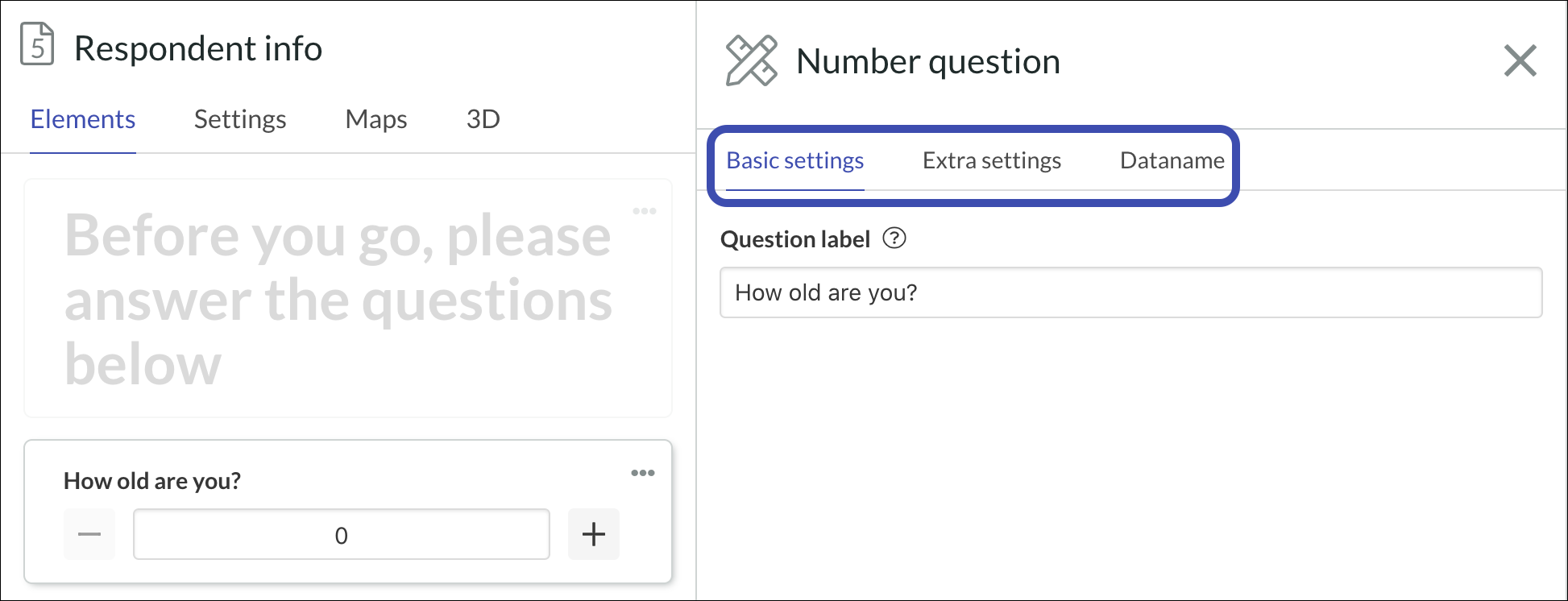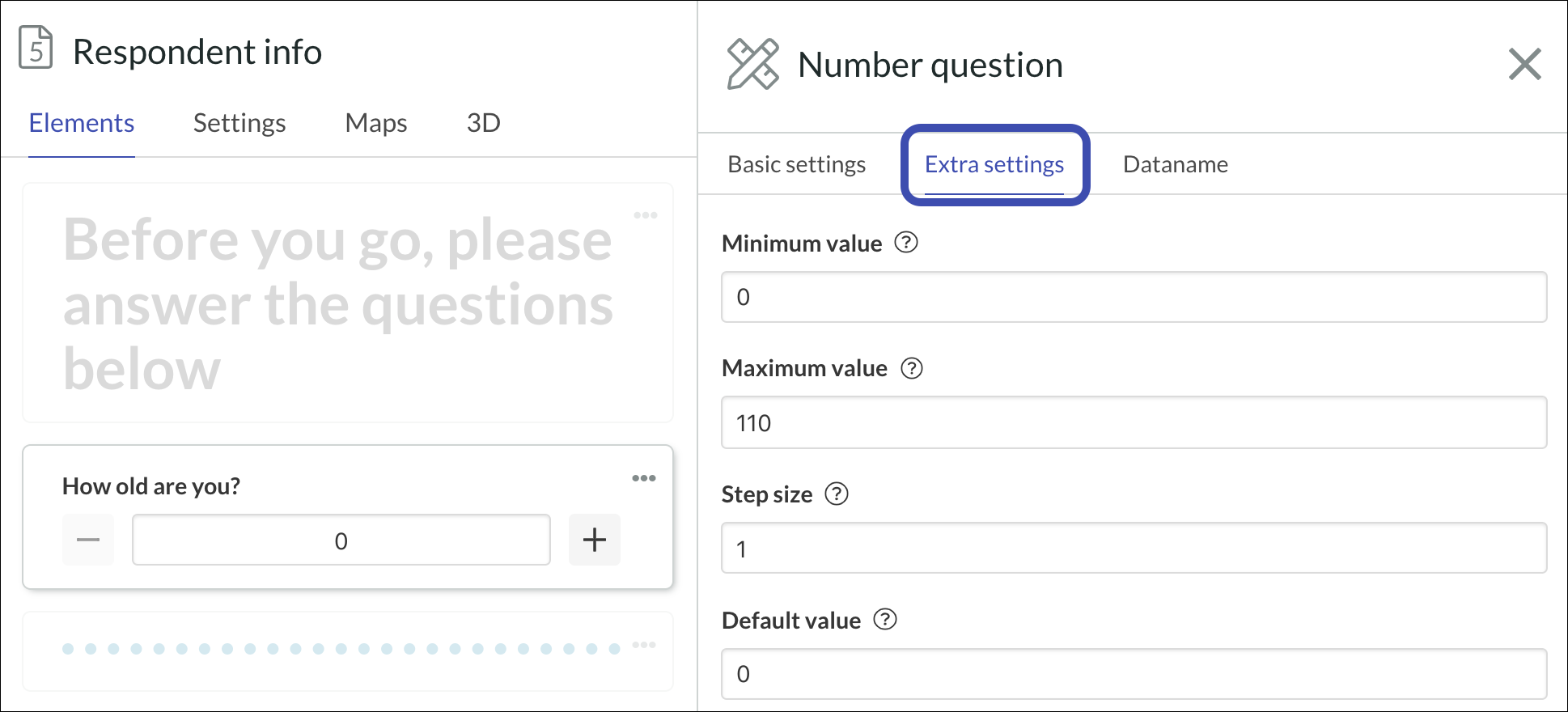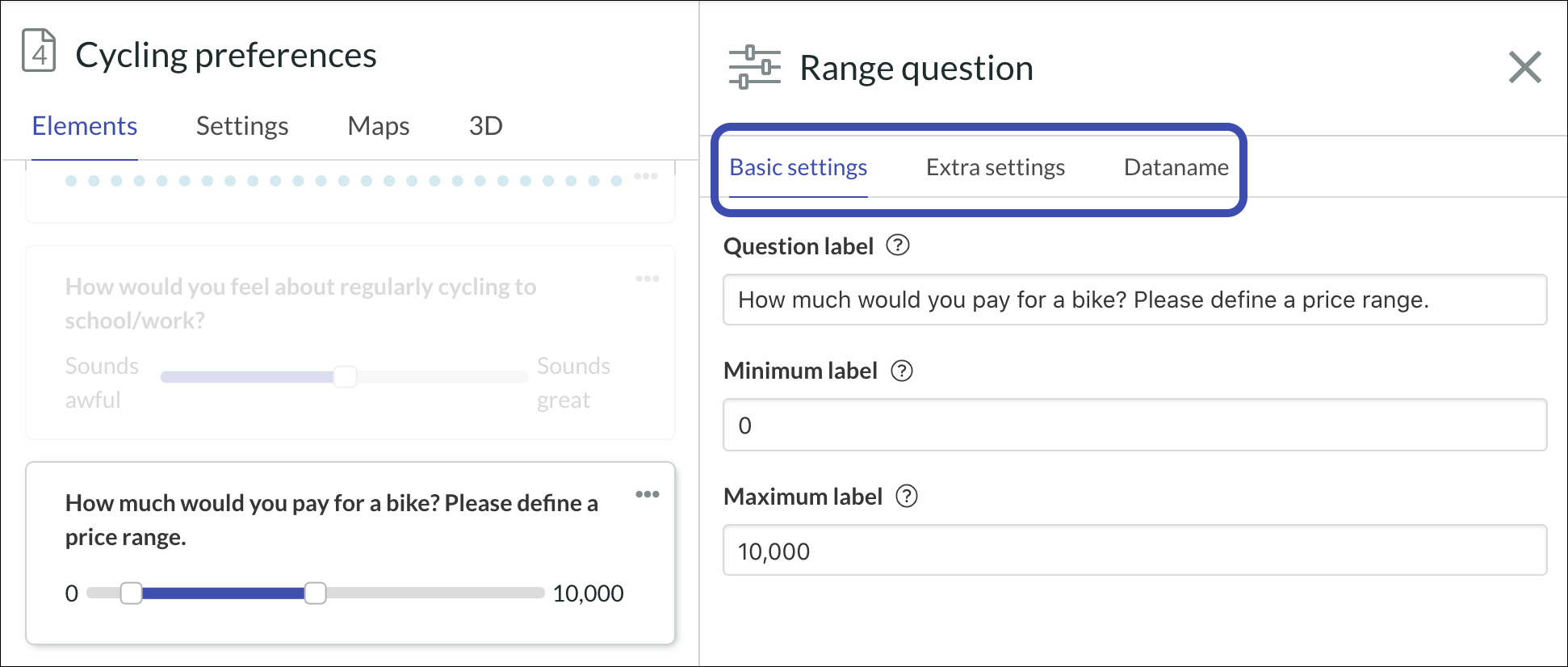# Number and range questions

Number question

This question element enables you to ask the respondent to answer with a number. The respondent uses the + and - signs to add or detract numbers.

How to use this element:

1. Like other elements, the number element's settings are also divided into Basic, Extra and Dataname. Go to the first tab and write the question in Question label.2. In Extra settings, you can choose:
• Minimum and maximum values that can be chosen by the respondent.
• The step size, which is the distance between each value.
• The default value, which is the value that will be shown in the value field when the respondent first uploads the page. In the example above, the value is 0.#### Asking for the respondent's birth year

If you want to ask your respondents for their birth year, you can use this question element for that purpose instead of a multiple-choice question. Respondents are able to type the year or value on the number field.

Range question

This question element enables you to ask the respondent to give a range of numbers as an answer. The respondent moves the two ends of the slider to define the range.

How to use this element:

1. Like other elements, the number element's settings are also divided into Basic, Extra and Dataname. First, go to the Basic settings tab and write  the question in Question label. Then, give the labels used for the maximum and minimum ends of poles values or titles.2. In the Extra settings, you can choose:

• The label position, which is the position of the pole labels.
• Whether you want to hide the value display on the range slider.
• The minimum and maximum values of the poles
• The step size, which is the distance between each value
• The default range, which will be visible when the respondent first uploads the page.